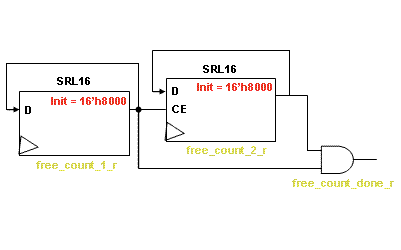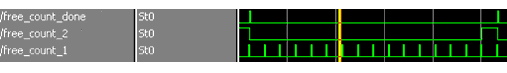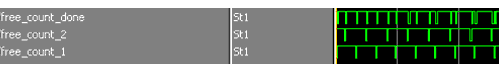# AR# 32218

|

## Description

Upon power-up, the Aurora Core does not always get initialized. When initialization fails, it continues to fail even if I assert the reset signal.

## Solution

To detect the problem during initialization, you should look at the lane up status when the core is in a failure state. If the lane up status toggles at a specific period of time, the issue is most likelydue to the "aurora_201_channel_init_sm.v[hd]" file.

In "aurora_201_channel_init_sm.v[hd]", a timer is created using two SRL16 shift registers:SRL16 Timer

Please check the following signals:

Free_count_1_r
Free_count_2_r
Free_count_done

If these are all fine, the signals exhibit the following behavior:good behavior

If the timer does not work, the signals could exhibit a behavior similar to that shown below:wrong behavior

This issue will be fixed in the 11.2 release of the core (v4.2). This temporary answer record is intended to support customers until the new code release.

The best solution is to replace the two series SRL16 with a simple counter that can be reset; see example code below.

VHDL:

1) Include the IEEE librarys STD_LOGIC_UNSIGNED package:

use IEEE.STD_LOGIC_UNSIGNED.all;

2) Add the following signal declaration:

signal free_count_r : std_logic_vector(0 to 7); --AR 32218 fix

3) Comment out the free_count_done_r assignment process. Example:

-- process (USER_CLK)

-- begin

-- if (USER_CLK 'event and USER_CLK = '1') then

-- free_count_done_r <= free_count_2_r and free_count_1_r after DLY;

-- end if;

-- end process;

4) Add the following counter code:

process (USER_CLK) --AR 32218 fix

begin

if (USER_CLK 'event and USER_CLK = '1') then

if ((RESET or RESET_CHANNEL) = '1') then

free_count_r <= (others => '0') after DLY;

else

free_count_r <= free_count_r + '1' after DLY;

end if;

end if;

end process;

free_count_done_r <= '1' when (free_count_r = "11111111") else '0'; --AR 32218 fix

Verilog:

1) Add the following reg declaration:

reg [0:7] free_count_r; //AR 32218 fix

2) Comment out the free_count_done_r assignment process. Example:

//always @(posedge USER_CLK)

// free_count_done_r <= `DLY free_count_2_r & free_count_1_r;

3) Add the following counter code:

always @ (posedge USER_CLK) //AR 32218 fix

if(RESET | RESET_CHANNEL)

free_count_r <= `DLY 8'b0000_0000;

else

free_count_r <= `DLY free_count_r + 1'b1;

always @ (posedge USER_CLK) //AR 32218 fix

free_count_done_r = (free_count_r == 8'b1111_1111);

AR# 32218
Date 09/18/2012
Status Active
Type General Article
Tools
IP
People Also Viewed